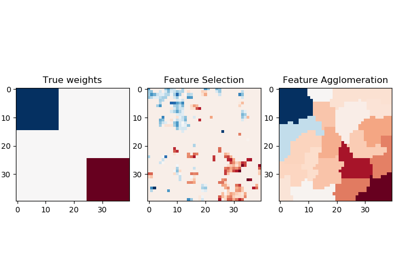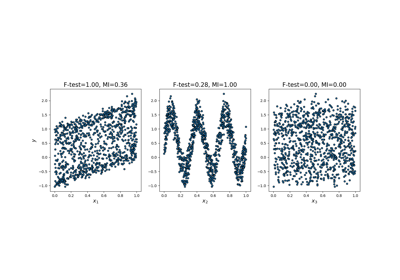sklearn.feature_selection.f_regression¶

sklearn.feature_selection.f_regression(X, y, center=True)[source]

Univariate linear regression tests.

Linear model for testing the individual effect of each of many regressors. This is a scoring function to be used in a feature selection procedure, not a free standing feature selection procedure.

This is done in 2 steps:

1. The correlation between each regressor and the target is computed, that is, ((X[:, i] - mean(X[:, i])) * (y - mean_y)) / (std(X[:, i]) * std(y)).
2. It is converted to an F score then to a p-value.

For more on usage see the User Guide.

Parameters: X : {array-like, sparse matrix} shape = (n_samples, n_features) The set of regressors that will be tested sequentially. y : array of shape(n_samples). The data matrix center : True, bool, If true, X and y will be centered. F : array, shape=(n_features,) F values of features. pval : array, shape=(n_features,) p-values of F-scores.

mutual_info_regression
Mutual information for a continuous target.
f_classif
ANOVA F-value between label/feature for classification tasks.
chi2
Chi-squared stats of non-negative features for classification tasks.
SelectKBest
Select features based on the k highest scores.
SelectFpr
Select features based on a false positive rate test.
SelectFdr
Select features based on an estimated false discovery rate.
SelectFwe
Select features based on family-wise error rate.
SelectPercentile
Select features based on percentile of the highest scores.

Examples using sklearn.feature_selection.f_regression¶Feature agglomeration vs. univariate selectionComparison of F-test and mutual informationPipeline Anova SVM